##### Actions

A quadratic form in four variables. A quaternary quadratic form over a fieldis related to the algebra of quaternions (cf. Quaternion) over the same field. Namely, corresponding to the algebra with basis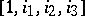,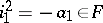,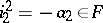, and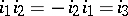, is the quaternary quadratic form which is the norm of the quaternion,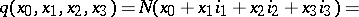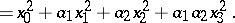For quaternary quadratic forms corresponding to quaternion algebras, and only for these, composition of quaternary quadratic forms is defined:where the coordinates of the vectorare bilinear forms inand. Composition of this kind is possible only for quadratic forms in two, four and eight variables.